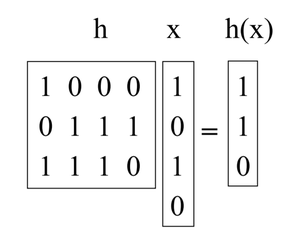# Introduction to Universal Hashing in Data Structure

• Last Updated : 19 Oct, 2021

Hashing is a great practical tool, with an interesting and subtle theory too. In addition to its use as a dictionary data structure, hashing also comes up in many different areas, including cryptography and complexity theory

This article discusses an important notion: Universal Hashing (also known as universal hash function families).

Attention reader! Don’t stop learning now. Get hold of all the important DSA concepts with the DSA Self Paced Course at a student-friendly price and become industry ready.  To complete your preparation from learning a language to DS Algo and many more,  please refer Complete Interview Preparation Course.

In case you wish to attend live classes with experts, please refer DSA Live Classes for Working Professionals and Competitive Programming Live for Students.

Universal Hashing refers to selecting a hash function at random from a family of hash functions with a certain mathematical property. This ensures a minimum number of collisions.

A randomized algorithm H for constructing hash functions h : U → {1,… ,M} is universal if for all (x, y) in U such that x ≠ y, Pr h∈H [h(x) = h(y)] ≤ 1/M (i.e, The probability of x and y such that h(x) = h(y) is <= 1/M for all possible values of x and y).

A set H of hash functions is called a universal hash function family if the procedure choose h ∈ H at random is universal. (Here the key is identifying the set of functions with the uniform distribution over the set.)

Theorem: If H is a set of the universal hash function family, then for any set S ⊆ U of size N, such that x ∈ U and y ∈ S, the expected number of collisions between x and y is at most N/M.

Proof: Each y ∈ S (y ≠ x) has at most a 1/M chance of colliding with x by the definition of “universal”. So,

• Let Cxy = 1 if x and y collide and 0 otherwise.
• Let Cx denote the total number of collisions for x. So, Cx = ∑ y∈S, x≠y Cxy.
• We know E[Cxy] = Pr[ x and y collide ] ≤ 1/M.
• So, by the linearity of expectation, E[Cx] = ∑y E[Cxy] < N/M.

Corollary: If H is a set of the universal hash function family, then for any sequence of L insert, lookup, or delete operations in which there are at most M elements in the system at any time, the expected total cost of the L operations for a random h ∈ H is only O(L) (viewing the time to compute h as constant).

For any given operation in the sequence, its expected cost is constant by the above theorem. Therefore, the expected total cost of the L operations is O(L) by the linearity of expectation.

Constructing a universal hash family using the matrix method:

Let’s say keys are u-bits long and the table size M is the power of 2, so an index is b-bits long with M = 2b.

What we will do is pick h to be a random b-by-u binary matrix, and define h(x) = hx, where hx is calculated by adding some of the columns of h (doing vector addition over mod 2) where the 1 bits in x indicate which columns to add. (e.g., the 1st and 3rd columns of h are added in the below example). These matrices are short and fat. For instance:Now, take an arbitrary pair of keys (x, y) such that x ≠ y. They must differ someplace, let’s assume they differ in the ith coordinate, and for concreteness say xi = 0 and yi = 1. Imagine we first choose all of h but the ith column. Over the remaining choices of the ith column, h(x) is fixed. However, each of the 2b different settings of the ith column gives a different value of h(y) (in particular, every time we flip a bit in that column, we flip the corresponding bit in h(y)). So there is exactly a 1/2b chance that h(x) = h(y).

My Personal Notes arrow_drop_up